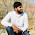Lewis Dot Structure of thiocyanate (SCN-) | Chemistry Net

# Lewis Dot Structure of thiocyanate (SCN-)

Lewis Dot Structure of thiocyanate ion (SCN-)

# Lewis Dot Structure of thiocyanate (SCN-)

A simple procedure for writing covalent Lewis Structures was given in a previous article entitled Lewis Structures and the Octet Rule”.

Several worked examples relevant to this procedure were given in previous posts please see the Sitemap - Table of Contents (Lewis Electron Dot Structures).

Let us consider the case of SCN-. Thiocyanate is the conjugate base of thiocyanic acid (HSCN). It is also known as rhodanide (from the Greek word for rose) because of the red color of its complexes with iron. Common salts include the colorless salts sodium thiocyanate and pottasium thiocyanate. It is produced by the reaction of elemental sulfur with cyanide:

8 CN + S8 → 8 SCN

The Lewis structures of thiocyanate are drawn below using the following four-step method:

Step 1: Connect the atoms with single bonds.Step 2: Calculate the # of electrons in π bonds (multiple bonds) using formula (1):

Where n in this case is 3. Where V = (6 + 4 + 5) – (-1) = 16 , V is the number of valence electrons of the molecule.

Therefore, P = 6n + 2 – V = 6 * 3 + 2 – 16 = 4 So, there are : 2 double bonds or a triple bond.

Step 3 & 4: The resonance structures of SCN- are as follows:Structure #1 is the most stable resonance Lewis structure since the octet rule is obeyed and the negative formal charge is carried out by N (electronegativity: 3.04) the most electronegative atom compared to S (electronegativity: 2.58). Structure #3 is less stable than #1 but more stable than #2 since the latter has the greatest charge separation. Therefore in terms of stability: resonance structure #1 > resonance structure #3 > resonance structure #2.

Relevant Posts

Lewis Structures|Octet Rule: A Simple Method to write Lewis Structures

Canonical Structures - Lewis Dot Structure of OCN-

References
1. G.N. Lewis, J.A.C.S, 38, 762-785, (1916)
2. E. C. McGoran, J. Chem. Educ., 68, 19-23 (1991)
3. A.B.P. Lever, J. Chem. Educ., 49, 819-821, (1972)

Key Terms

lewis structures, simple method for writing Lewis structures, valence electrons, single bonds, electrons in π bonds, octet rule,most stable resonance structure, resonance structures, electronegative atom, formal charge,

#### 1 comment:

1.